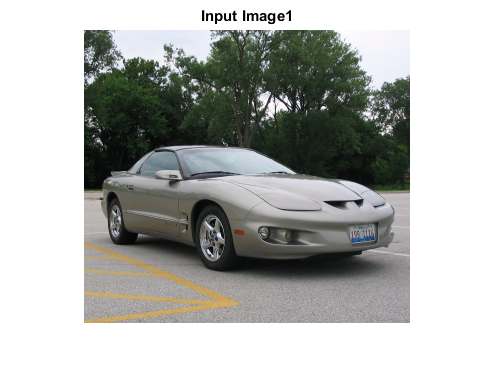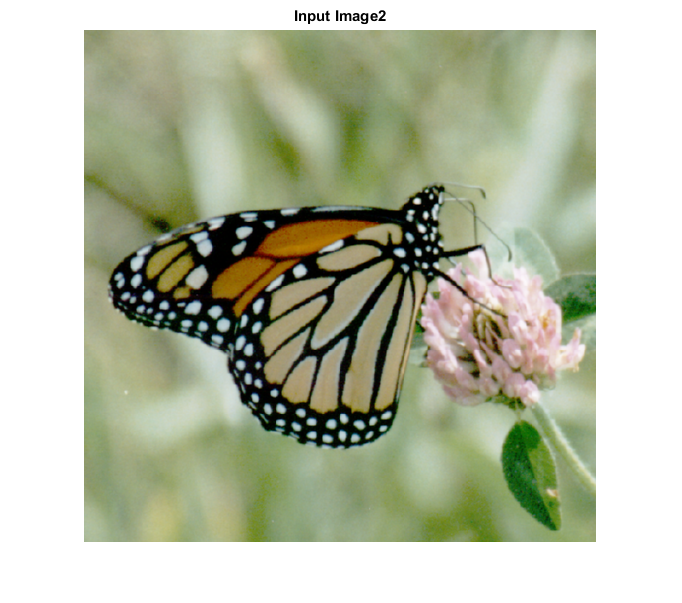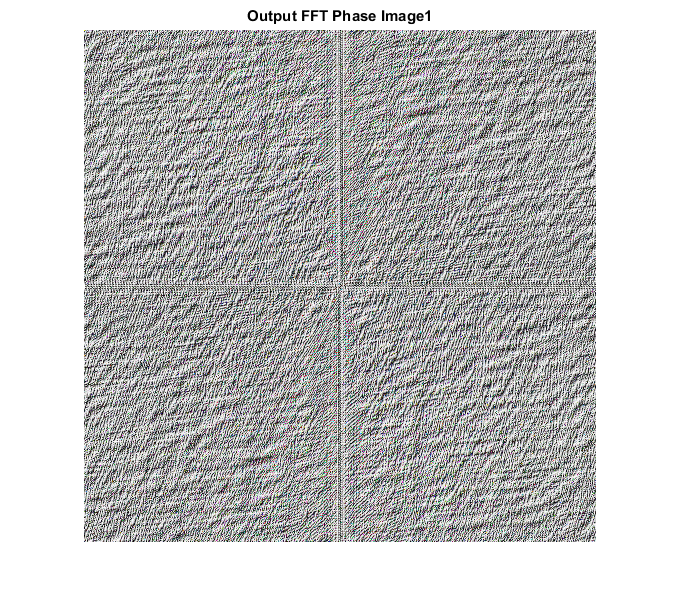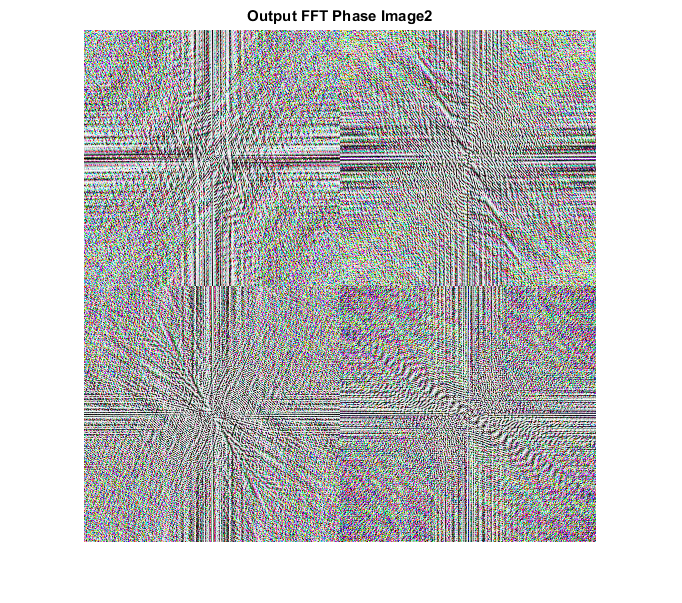# FFT_PHASE_CVIP

fft_phase_cvip() - extracts phase of the Fourier spectrum.

## SYNTAX

`phase = fft_phase_cvip( input_img, block_size )`

Input Parameters include :

• input_img - The orignial image which can be grayscale or RGB.
• block_size - Should be a power of 2 or [].if empty, then functions finds the nearest power of 2 and zero pad the image, to that block size.Any other value smaller than image size will partiotion the image to windows of that block size and FFT will be calculated separately in each window.

Output Parameters include :

• Phase - Phase of the FFT.

## DESCRIPTION

The function performs an FFT on the input image of a block size specified by the user and returns only the Phase information of the resultant spectrum.

## REFERENCE

1. Scott E Umbaugh. DIGITAL IMAGE PROCESSING AND ANALYSIS: Applications with MATLAB and CVIPtools, 3rd Edition.

## EXAMPLE

```% Read image

% Block size

block_size1 = [];

block_size2 = 256;

% Call function

spect1 = fft_phase_cvip( input_img1, block_size1 );

spect2 = fft_phase_cvip( input_img2, block_size2 );

% use abs to compute the magnitude (handling imaginary) and use log to brighten display

S1=log(1+abs(spect1));

S1 = remap_cvip(S1);

S2=log(1+abs(spect2));

S2 = remap_cvip(S2);

% Display input image

figure; imshow(input_img1);title('Input Image1');

figure;imshow(input_img2);title('Input Image2');

% Display output image

figure; imshow(S1,[]);title('Output FFT Phase Image1');

figure;imshow(S2,[]);title('Output FFT Phase Image2');
```